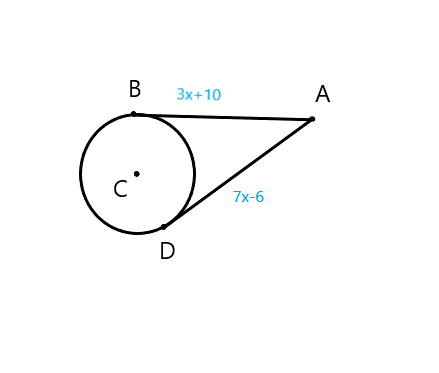Ask question

# Point K is on line segment ‾JL. Given JK = 2x - 2, KL = x - 9, and JL = 2x + 8, determine the numerical length of ‾KL.# Point K is on line segment ‾JL. Given JK = 2x - 2, KL = x - 9, and JL = 2x + 8, determine the numerical length of ‾KL.

Question
Analytic geometryasked 2021-02-01

Point K is on line segment $$\overline{JL}$$. Given $$JK = 2x - 2, KL = x - 9,\ and\ JL = 2x + 8,$$ determine the numerical length of $$\overline{KL}$$.

## Answers (1)2021-02-02

By Angle Addition Postulate,
$$JL=JK+KL$$
Substitute given expressions:
$$2x+8=(2x−2)+(x−9)$$
Solve for x:
$$2x+8=3x−11$$
$$−x+8=−11$$
$$−x=−19$$
$$x=19$$
Hence,
$$KL=19−9$$
$$KL=10$$

### Relevant Questionsasked 2021-01-04

Consider rectangel CREB that is formed of squares COAB and OREA, where $$AE=4ft$$. Assume squares COAB and OREA are congruent.
$$BO=3m-2n$$
$$AR=7(m-1)-4.8n$$
Determine the values of m and n and the length of RB.asked 2021-01-10

$$\displaystyle{\cos{{2}}}\theta=\frac{{28}}{{53}}$$
Find $$\displaystyle{\sin{\theta}}$$, if
$$\displaystyle{0}^{\circ}{<}\theta{<}{90}^{\circ}$$asked 2020-10-26

Determine the value of k for which the point (- 2, 3) lies on the line whose equation is .

$$4x+3ky=10$$asked 2020-12-16

The gazebo in the photo is built in the shape of a regular octagon. Each side is 13ft long and the enclosed area is $$816.4ft^2$$
What is the length of the apothem?asked 2021-03-29
Two stationary point charges +3 nC and + 2nC are separated bya distance of 50cm. An electron is released from rest at a pointmidway between the two charges and moves along the line connectingthe two charges. What is the speed of the electron when it is 10cmfrom +3nC charge?
Besides the hints I'd like to ask you to give me numericalsolution so I can verify my answer later on. It would be nice ifyou could write it out, but a numerical anser would be fine alongwith the hint how to get there.asked 2021-05-27

Use the given conditions to write an equation for the line in point-slope form and in slope-intercept form.
Passing through (-2,-8) and parallel to the line whose equation is $$y=-3x+4$$
Write an equation for the line in point-slope form and slope-intercept form.asked 2021-05-19
For digits before decimals point, multiply each digit with the positive powers of ten where power is equal to the position of digit counted from left to right starting from 0.
For digits after decimals point, multiply each digit with the negative powers of ten where power is equal to the position of digit counted from right to left starting from 1.
1) $$10^{0}=1$$
2) $$10^{1}=10$$
3) $$10^{2}=100$$
4) $$10^{3}=1000$$
5) $$10^{4}=10000$$
And so on...
6) $$10^{-1}=0.1$$
7) $$10^{-2}=0.01$$
8) $$10^{-3}=0.001$$
9) $$10^{-4}=0.0001$$asked 2021-04-06
$$\displaystyle{Q}={\int_{{4}}^{{9}}}{\frac{{{3}{x}+{12}}}{{{x}^{{2}}+{6}{x}+{9}}}}{\left.{d}{x}\right.}$$
Determine the numerical values of the coefficients, A and B, where $$\displaystyle{A}\leq{B}$$.
$$\displaystyle{\frac{{{A}}}{{{d}{e}{n}{o}{m}{i}{a}{n}{a}\to{r}}}}+{\frac{{{B}}}{{{d}{e}{n}{o}{m}{i}{a}{n}\to{r}}}}$$
Need to find A and B.asked 2021-05-08

Points B and D are points of tangency. Find the value of x..asked 2021-04-13
As depicted in the applet, Albertine finds herself in a very odd contraption. She sits in a reclining chair, in front of a large, compressed spring. The spring is compressed 5.00 m from its equilibrium position, and a glass sits 19.8m from her outstretched foot.
a)Assuming that Albertine's mass is 60.0kg , what is $$\displaystyle\mu_{{k}}$$, the coefficient of kinetic friction between the chair and the waxed floor? Use $$\displaystyle{g}={9.80}\frac{{m}}{{s}^{{2}}}$$ for the magnitude of the acceleration due to gravity. Assume that the value of k found in Part A has three significant figures. Note that if you did not assume that k has three significant figures, it would be impossible to get three significant figures for $$\displaystyle\mu_{{k}}$$, since the length scale along the bottom of the applet does not allow you to measure distances to that accuracy with different values of k.
...